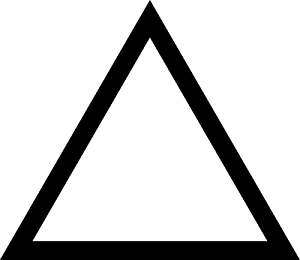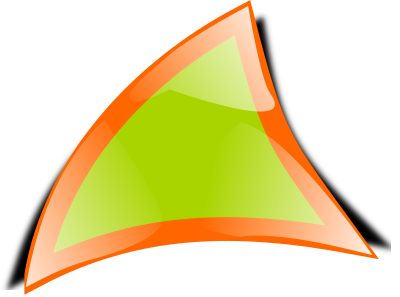# Area of a Triangle

Instructions: Use this calculator to find the area of a triangle, with a given the base b and the height h. Please provide the base and height in the boxes below.The base $$b$$ of the triangle (Ex: 2, or 3/2, etc.)The height $$h$$ of the triangle (Ex: 2, or 3/2, etc.)

This calculator will compute the area of a triangle, for a given base $$b$$ and height $$h$$ that you provide. The provided base and height of the triangle must be valid numeric expressions. You can type in number, using decimals or not, you can type in fractions, or any valid algebraic expressions like '4/3', or 'sqrt(5)', etc.

Once you have provided the required information to construct the triangle, all you need to do is to click on "Calculate", and all the steps of the calculation of the area will be shown, as well as a graph showing a graphical depiction of the corresponding triangle.

There are times where you don't have directly the base and the height. There are cases where you need to compute the area and you have the three sides. Or you could have two sides and one angle or two angles and one side.## How do compute the area of a rectangle?

So the actual should be what is the formula to compute the area of a triangle. The answer is simple and it is essentially based on multiplying base and height together and then dividing by 2. So, if the based and the height $$b$$ and $$h$$, then the formula for the area of the triangle is

$\text{Area} = \displaystyle \frac{b h}{2}$

## What are the steps for computing the area of a triangle

• Step 1: Identify clearly the based and height of the triangle being provided, and call them 'b' and 'h' respectively
• Step 2: Once you know the the base and height 'b' and 'h', the area is computed b*h/2
• Step 3: If needed, identify the units of 'b' and 'h' and give units to the area

Observe that a part of Step 1 consists on assessing whether or not you have the base and height, otherwise you need to use other methods to compute the area, as explained in the previous section.

## What do you use the area of the triangle for?

Triangles were one of the most profusely studied geometric shapes, and it has a countless number of incredible properties. The Greek indeed proved a myriad of theorems about triangles, that make them a REALLY interesting object of study.

In practice, a whole lot of application include the use of triangles, and they along with squares, rectangles and circles are the must-know geometric shapes for you.### Example: Calculate the area of a rectangle

Calculate the area of rectangle with given based of b = 3 and height h = 5.

Solution: We first need to identify whether or not the base and height are provided (otherwise a different method would need to be used). In this case, it is clearly specified that the base is b = 3 and the height is h = 5. Then, the appropriate formula for the area is:

$\text{Area} = \displaystyle \frac{b h}{2}$

Then, we need to plug in the values b = 3 and h = 5 into the formula:

$Area = \displaystyle \frac{b h}{2} = \displaystyle \frac{3 \times 5}{2} = 7.5$

so the area of the given triangle is 7.5.

### Example: Area of a triangle with length units

Calculate the area of triangle with base of b = 3.2 cm and height h = 5 cm

Solution: As in the previous case, the first thing to do is to identify the base and height, which in this case are b = 3.2 cm and h = 5 cm. We have length units (cm) in this case. The formula for the area is the same as before:

$Area = \displaystyle \frac{b h}{2} = \displaystyle \frac{3.2 \times 5}{2}\, cm^2 = 16 \, cm^2$

which indicates that the area is 16 cm2.

## More area calculators

There are as many area calculators as geometric shapes that you can think of. Other shapes you may be interested are the area of a rhombus and the area of a square as well as the area of the rectangle. All of them use very similar methods for the derivation.

For shapes that don't have straight sides, we can use this area of circle calculator and this area of an ellipse calculator.